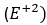# Chemical reactivity with halogens of Group 1 and Group 2 elements

Group 1:

The elements belonging to group 1 are called alkali metals. They constitute the six elements namely, lithium(Li), sodium(Na), potassium(K), rubidium(Rb), cesium(Cs) and francium(Fr). These elements are called alkali metals because they readily dissolve in water to form hydroxides which are strongly alkaline in nature. They also from alkaline oxides. The element, francium is radioactive.

Reactivity with halogens:

The alkali metals readily react with halogens to form ionic halides of the form $M^{+}X^{-}$

Have a look at the reaction here: $2M+ X_{2} \to 2MX$ (X=Halogens)

$2Na+ Cl_{2} \to 2NaCl$

$2K+ Cl_{2} \to 2KCl$

• The reactivity of alkali metals towards a particular halogen increases on moving down the group. This is due to a decrease in ionization enthalpy or an increase in electropositive character as we move down the group.
• All the metal halides are ionic crystals. However, lithium iodide LiI is slightly covalent because of the polarization ($Li^{+}$) being the smallest cation and has maximum polarizing power and iodide ion being the largest anion can be polarized to the maximum extent.
• The alkali metal halides are high melting colorless crystalline solids. All these halides have high negative enthalpies of formation. The enthalpies of formation for fluorides become less negative as we go down the group while the reverse is true for the enthalpies of formation of chlorides, bromides, and iodides. Thus, the fluorides of alkali metals are most stable while iodides of alkali metals are least stable.
• All the alkali metal halides are soluble in water except LiF. However, LiF is insoluble in water due to its high lattice energy because of the small size of cation and anion.

Group 2:

Group 2 elements are known as alkaline earth metals. The group 2 consists of the elements beryllium(Be), magnesium(mg), calcium(Ca), strontium(Sr), barium(Ba) and radium(Ra).

They have a low enthalpy of ionization and high electropositive character, the alkaline earth metals have a strong tendency to lose valence electrons. Therefore, they are very reactive. The reactivity of these elements increases on going down the group. However, alkaline earth metals are generally less reactive than alkali metals. This is because of their dipositive oxidation stateReactivity with halogens:

All the alkaline earth metals combine with halogens at higher temperatures forming their halides as shown here: $M+ X_{2} \to MX_{2}$ (X = F, Cl, Br, I)

The metal halides can also be obtained by the action of halogen acids on metals, their oxides, carbonates, and hydroxides. All the reactions are shown below:

$MO+2HX \to MX_{2}+ H_{2}O$

$M(OH)_{2}+2HX \to MX_{2}+ 2H_{2}O$

Be$F_{2}$ is conveniently prepared by the thermal decomposition of $(NH_{4})_{2}BeF_{4}$ while BeC$l_{2}$ can be made from its oxide as shown here:

$BeO+2NH_{3}+ 4HF \to (NH_{4})_{2}\lbrack BeF_{4}\rbrack \to BeF_{2}+ 2NH_{4}F$

$BeO+C+ Cl_{2} \leftrightarrow BeCl_{2}+ CO$

Beryllium chloride can also be prepared by heating beryllium oxide with carbon tetrachloride as shown in the reaction below:

$2BeO+CCl_{4} \to 2BeCl_{2}+ CO_{2}$

The anhydrous halides of alkaline earth metals are hygroscopic, they absorb moisture from air and form hydrates as $BeCl_{2} . 4H_{2}O, CaCl_{2} . 6H_{2}O, MgCl_{2 }. 6H_{2}O.$ This tendency of forming halide hydrates gradually decreases down the group from Mg to Ba. Except for the beryllium and magnesium chloride, the chlorides of other members of the group 2 imparts characteristic color to the flame.